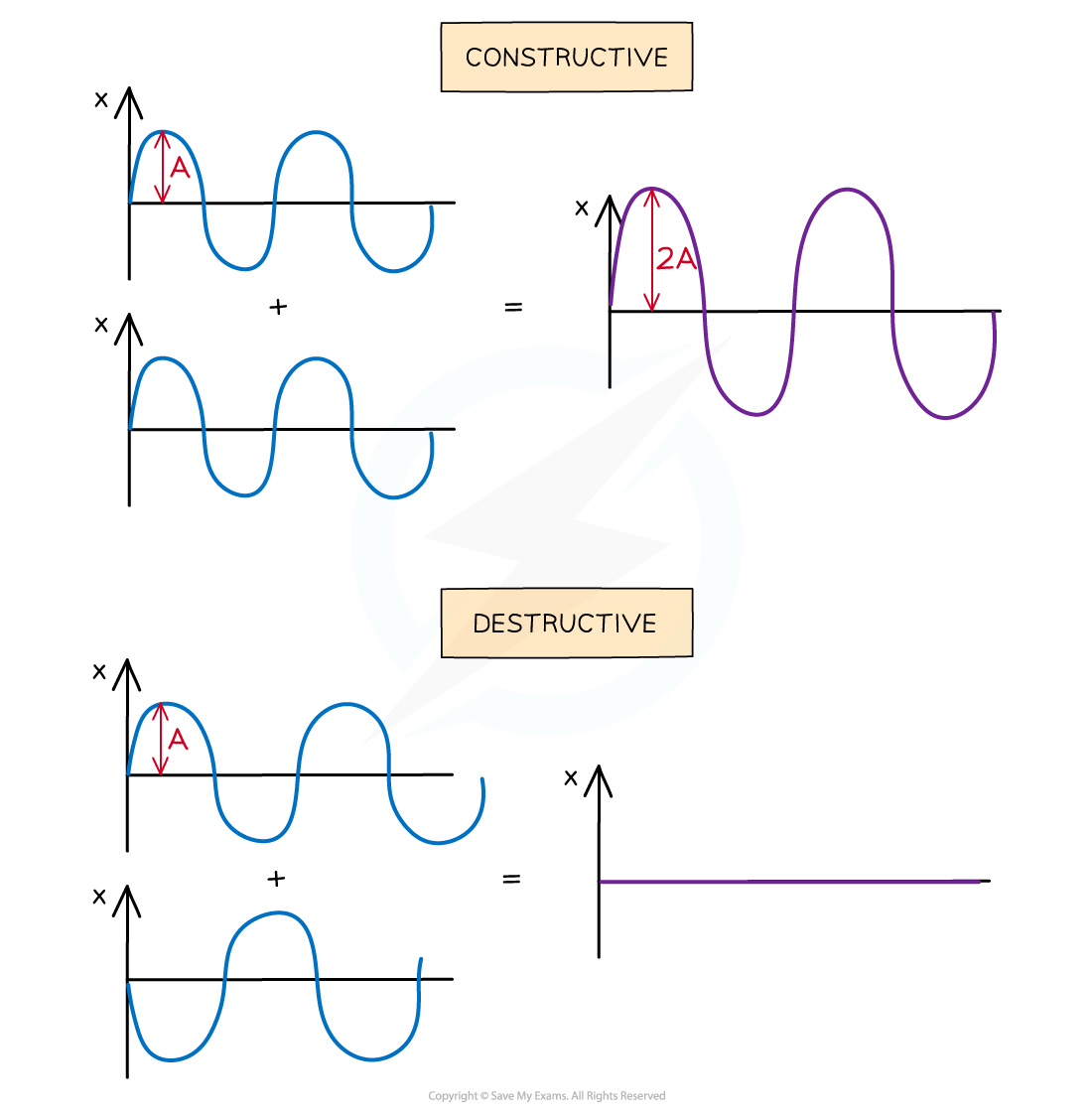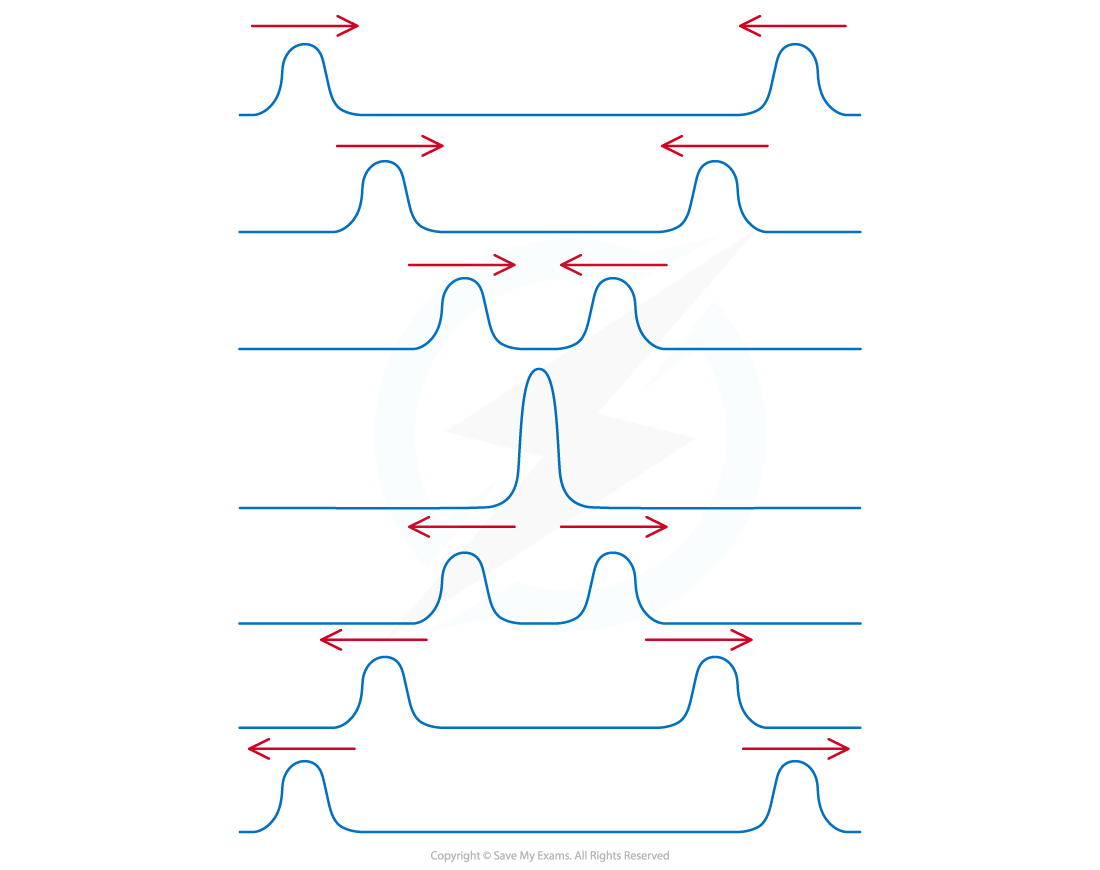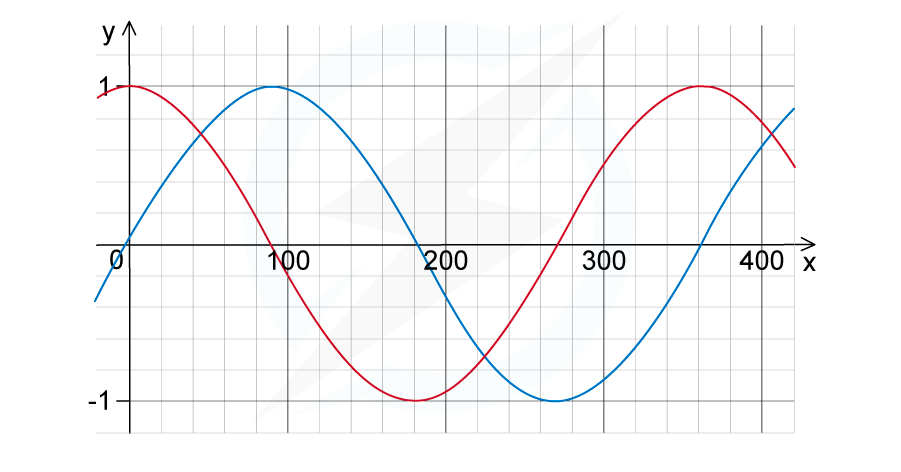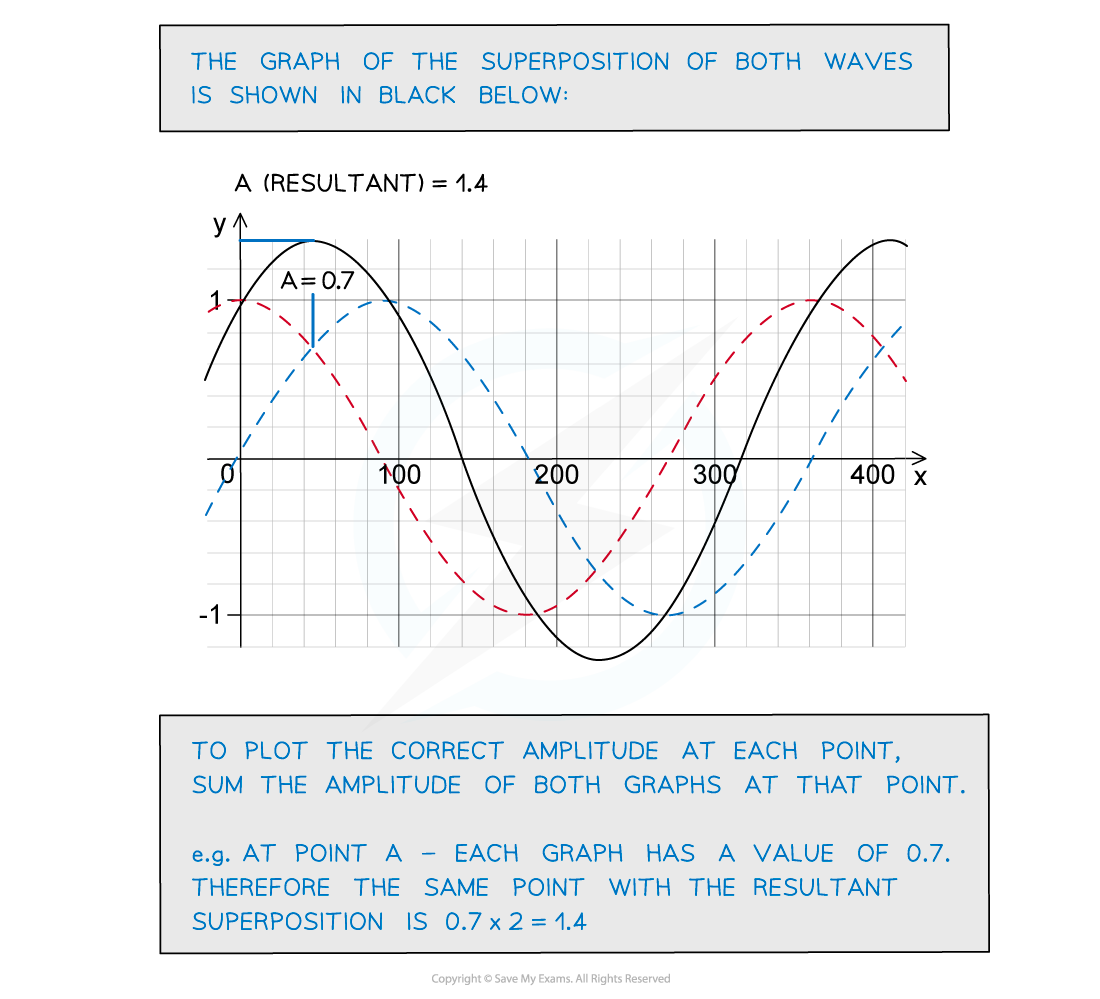# IB DP Physics: SL复习笔记4.3.3 Superposition

### Superposition

• The principle of superposition states that:

When two or more waves meet, the resultant displacement is the vector sum of the displacements of the individual waves

• The principle of superposition applies to both transverse and longitudinal waves
• Interference occurs whenever two or more waves superpose
• For a clear stationary interference pattern, the waves must be of the same:
• Type
• Amplitude
• Frequency
• They must also have a constant phase difference

#### Constructive & Destructive Interference

• Constructive interference occurs when the waves superpose and have displacements in the same direction (both positive or both negative)
• Destructive interference occurs when the waves superimpose and have displacements in opposite directions (one positive and one negative)
• When two waves with the same amplitude meet at a point, they can:
• Be in phase and interfere constructively, so that the displacement of the resultant wave is double the displacement of each individual wave
• Be in anti-phase and interfere destructively, so that the displacement of the resultant wave is equal to zeroWaves in superposition can undergo constructive or destructive interference

• Superposition occurs for any two waves or pulses that overlap, and can result in a mix of constructive and destructive interference
• For example, the peak of one wave superposes with the peak of another wave with a smaller displacement
• The resultant peak will have a displacement that is in the middle of the displacement of both waves
• Superposition can also be demonstrated with two pulses
• When the pulses meet, the resultant displacement is the algebraic sum of the displacement of the individual pulses
• After the pulses have interacted, they then carry on as normal#### Worked Example

Two overlapping waves of the same types travel in the same direction. The variation with x and y displacement of the wave is shown in the figure below.Use the principle of superposition to sketch the resultant wave.